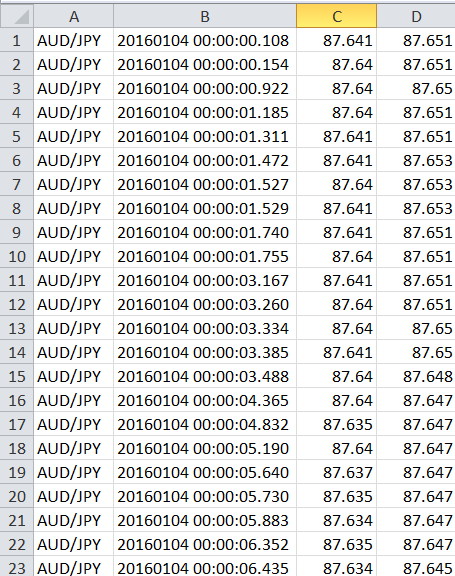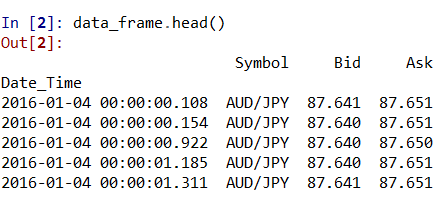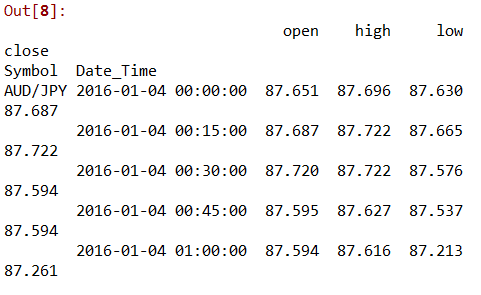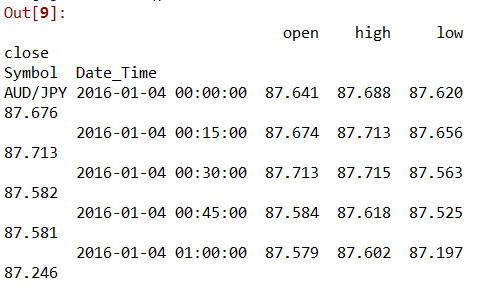# Converting Tick-By-Tick Data To OHLC Data Using Pandas Resample

In this post, we will explore a feature of Python pandas package. We usually find queries about converting tick-by-tick data into OHLC (Open, High, Low and Close) frequently. This can be accomplished with minimal effort using pandas package. The OHLC data is used for performing technical analysis of price movement over a unit of time (1 day, 1 hour etc.). We have already seen How OHLC data is used to calculate pivot points which traders use to identify key areas where reversal of price movement is possible, using which they can ideate their investment strategy.## The First Step

The first step involves fetching sample data. Let us download sample tick by tick data. For this tutorial, we will use the January data for AUD/JPY (Australian Dollar/Japanese Yen) pair that was downloaded from Pepperstone.

Pepperstone provides free historical tick data for various currency pairs. The .csv file contains top of the book, tick-by-tick market data, with fractional pip spreads in millisecond details. This data is more than sufficient for our analysis.## Steps In Python

As you can see the data is without any header. We will include the header and accomplish the required task programmatically.

### Import pandas package

import pandas as pd

Data is stored in my working directory with a name 'AUDJPY-2016-01.csv'. As we saw earlier, the data is without a header. Hence we would add header to the data while importing it. Thus importing and adding header take place in the same line of code.

data_frame = pd.read_csv('AUDJPY-2016-01.csv', names=['Symbol', 'Date_Time', 'Bid', 'Ask'],

index_col=1, parse_dates=True)
data_frame.head()

This is how the data frame looks like:-We use the resample attribute of pandas data frame. The resample attribute allows to resample a regular time-series data. We shall resample the data every 15 minutes and divide it into OHLC format. If you want to resample for smaller time frames (milliseconds/microseconds/seconds), use L for milliseconds, U for microseconds, and S for seconds.

data_ask = data_frame['Ask'].resample('15Min').ohlc()

data_bid =data_frame['Bid'].resample('15Min').ohlc()

A snapshot of tick-by-tick data converted into OHLC format can be viewed with the following commands:-

data_ask.head()

data_bid.head()You may concatenate ask price and bid price to have a combined data frame

data_ask_bid=pd.concat([data_ask, data_bid], axis=1, keys=['Ask', 'Bid'])

## The Complete Code

import pandas as pd
index_col=1, parse_dates=True)
data_bid =  data_frame['Bid'].resample('15Min').ohlc()
data_ask_bid=pd.concat([data_ask, data_bid], axis=1, keys=['Ask', 'Bid'])